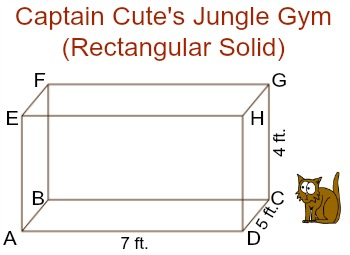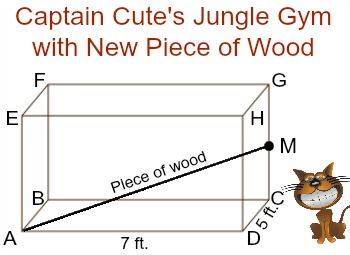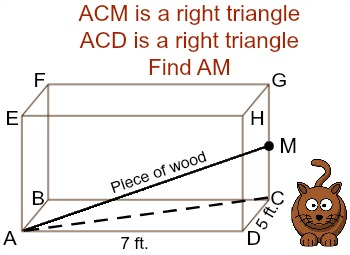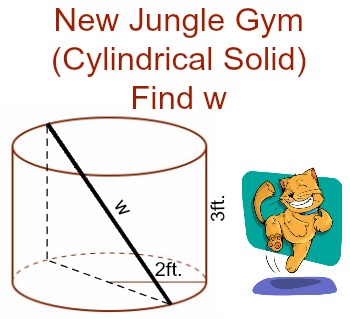# How to Find the Distance Between Points on a Solid

Instructor: Laura Pennington

Laura received her Master's degree in Pure Mathematics from Michigan State University, and her Bachelor's degree in Mathematics from Grand Valley State University. She has 20 years of experience teaching collegiate mathematics at various institutions.

This lesson will review the definitions of a solid, of a right triangle, and of the Pythagorean Theorem. Then we will look at how to use these concepts to find the distance between two points on a solid.

## Two Points on a Solid

Suppose you are building a jungle gym for your cat, Captain Cute, to play on. You've already put together a wood structure that has the shape of a large rectangular box with length 7 ft., width 5 ft., and height 4 ft.So far your jungle gym is what's called a solid in mathematics. A solid is a three-dimensional object, such as a sphere, a cylinder, or as in Captain Cute's case, a box (or a rectangular solid).

You decide that you want to add on a piece of wood from point A to the midpoint of CG, call it M, so that the Captain can get to the top of the structure easier.In order to do this, you need to know how long the piece of wood needs to be. In other words, we want to find the distance between points A and M on the solid. Yay! A math problem! Let's figure out how to do this!

## Distance Between Two Points on a Solid

Finding the distance between two points on a solid isn't too hard as long as we're familiar with right triangles and the Pythagorean Theorem. Right triangles are triangles that contain a right angle (90-degree angle). The two shorter sides of a right triangle are called the legs of the triangle, and the longest side is called the hypotenuse.

In a right triangle, if the shorter sides have lengths a and b, and the hypotenuse has length c, then the Pythagorean Theorem relates the lengths of these sides together using the following formula:

• a2 + b2 = c2

Now, let's look at why it is important to be familiar with these concepts when dealing with two points on a solid.

Notice that the piece of wood creates the right triangle ACM, where AM is the new piece of wood.We know the length of the side CM is one-half the length of CG, because M is the midpoint of CG. Therefore, CM = 2ft. Also notice that the side AC is the hypotenuse of the right triangle ACD, where AD = 7ft. and CD = 4ft., so we can find AC using the Pythagorean Theorem:

• AD2 + CD2 = AC2We get that AC = √65 ft..

Now we have the lengths of two of the sides of the right triangle ACM, so we can use the Pythagorean Theorem to find the length of our piece of wood, AM.

• AC2 + CM2 = AM2Awesome, we have that the piece of wood will be √69 ≈ 8.3 ft.. Captain Cute is going to be so happy!

## Another Example

As we just saw, finding the distance between two points on a solid is just a matter of using right triangles and the Pythagorean Theorem. That is, to find the distance between two points on a solid, we follow these steps:

1. Create a right triangle with one of its sides connecting the two points you want to find the distance between.
2. Use properties of the solid, other right triangles, and the Pythagorean Theorem to ultimately find the distance between your two points.

For example, suppose that after seeing how much fun Captain Cute has been having on his jungle gym, you decide to make another one. This one is a wood structure in the shape of a cylindrical solid with height 3 ft. and radius 2 ft. Once again, you want to add another piece of wood from the edge of the bottom base to the edge of the top base, and you need to know how long that piece of wood will be.To unlock this lesson you must be a Study.com Member.

### Register to view this lesson

Are you a student or a teacher?

Back

### Resources created by teachers for teachers

Over 30,000 video lessons & teaching resources‐all in one place.Video lessonsQuizzes & WorksheetsClassroom IntegrationLesson Plans

I would definitely recommend Study.com to my colleagues. It’s like a teacher waved a magic wand and did the work for me. I feel like it’s a lifeline.

Jennifer B.
TeacherCreate an account to start this course today
Used by over 30 million students worldwide